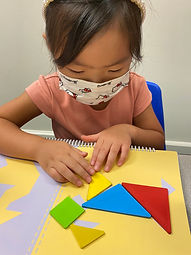## Ms. Brianne

### Target 1​

###### Lesson Type:

Continuation

Number Operation

:

Number System

Indicate the ordinal position of a given figure.

###### 1:

Use numbers to depict order and placement.

###### 2:

Use the three suffixes that turn numbers ordinal (-th, -rd, and -nd).

Kindergarden

###### Vocabulary:

Order, Position, Ordinal Numbers

Activities:

• Students created a line of 10 different animal counters and then wrote the ordinal numbers for each animal's position. Then they orally named the ordinal position for each animal.
• Students were given a line up of 10 pictures and wrote the ordinal number to describe each picture's position.### Home Exploration

###### Guiding Questions:## Absent Students:

### Target 2

:

###### 1:

Identify basic 2D shapes and their defining attributes (rhombus, parallelogram, hexagon, and octagon).

###### 2:

Identify the number of sides, number of corners (vertices), and side lengths of shapes.

1st

###### Vocabulary:

2D shape, Rhombus, Octagon, Hexagon, Pentagon, Sides, Corners

Activities:

• Students reviewed the 2D shapes they knew and were introduced to a rhombus, pentagon, hexagon, and octagon. For each shape, they identified the numbers of sides and corners.
• Students constructed 2D geometric shapes using straws.### Home Exploration

###### Guiding Questions:### Target 3

:

###### 1:

Solve simple tangram puzzles by recognize which spaces in the puzzle the given seven shapes can and cannot fit.

2nd

###### Vocabulary:

Tangrams, Triangles, Square, Parallelogram

Activities:

• Students learned about tangrams, and were introduced to the shape of a parallelogram.
• Students used visual and spatial reasoning to solve animal tangram puzzles. They used flips, slides, and turns to try to complete the puzzle.### Home Exploration